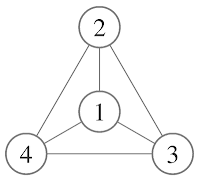## Wednesday, August 20, 2008

### The Balloon TetrahedronThe first balloon design is this 1-balloon tetrahedron design. It's not the easiest design but it's the easiest shape. The tetrahedron is composed of four triangles but I like to focus on the edges and vertices (I like to think in terms of graph theory). There are six edges and four vertices. The problem with the making the tetrahedron is that the vertices have degree three--this means that every vertex has a problem (see Eulerian path). A balloon comes into a vertex and leaves the vertex which takes care of two of the three edges but the remaining one has to be dealt with. So you can either have the balloon start or end at that vertex (but each balloon only has two ends) or you could cheat. There a number of ways to cheat but the one used here is to join each balloon with an interior point. With this design, each of the vertices now has degree four.

As well as a video of the construction, I'll include a diagram and a sequences of that describes the design similar to Gauss code. This is perhaps more to introduce the method of description rather than to be useful in this case. So here I'll have a planar graph with the vertices labeled but the interior point(0) omitted (which would make it non-planar).0z, 1, 2, 3, 1, 4, 2, 0, 4, 3, 0z

The z superscript indicates that the 0 is a delayed connection until the z subscript.